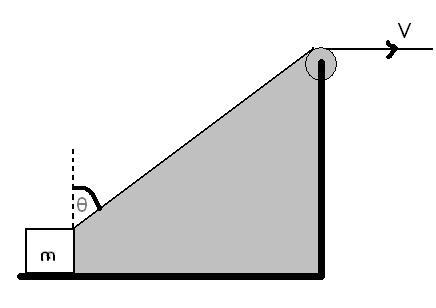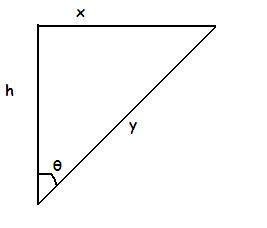# kinematicsA block is dragged on a smooth plane with the help of a rope which moves with velocity v shown in Fig. The horizontal velocity of the block is

a)v

b) vsinÎ¸

c) v/sinÎ¸

d) v/cosÎ¸

•Subhomoy Bakshi ·

v/sin Î¸

•Ashish Kothari ·

pls explain.

•Subhomoy Bakshi ·well from triangle we can say x=√y2-h2

so, dxdt=2y2√y2-h2.dydt

also dydt=v

so vx=v.(yy2-h2)=v.yx=vsin Î¸

sin Î¸= yx

•AKHIL ·

yes it is v/sin theta

•mohit sengar ·

can anyone tell me that how can we approach that it is a type of constraint relation.
i want to know the basic approach

•Subhomoy Bakshi ·

the basic approach is v=ds/dt wer s= displacement

and a=dv/dt

thus if we can some how relate the displacements of the particles we can relate their velocities and accelerations!! :)

•Vivek @ Born this Way ·

Waah!! You just told him the whole secret!

•Subhomoy Bakshi ·

LOL!!

exactly that's the secret!!

•Debayan Singha ·

what is so tricky about it

•kartu namak ·

i easily explained yesterday to my friend yesterday that answer is v/sintheta but today after 22 hrs i forgot myself that how my answer came yesterday

•Anuj Kumar ·

The component of block's velocity along the rope is equal to v.

v'sin(theta)=v (v' is velocity of block)

this is due to the fact that the string is unstretchable this can be proven by constaint relations or power method.

•